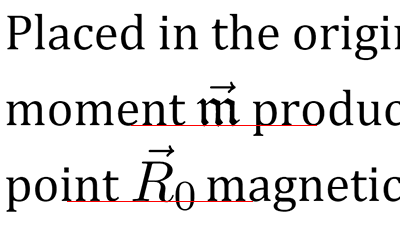# LaTeX equations for web

## Online editorImage URL:

## Examples

### Integrals, roots and borders

$$\boxed{ \int\limits_{-\infty}^{\infty} e^{-x^2} \, dx = \sqrt{\pi} }$$
\boxed{ \int\limits_{-\infty}^{\infty} e^{-x^2} \, dx = \sqrt{\pi} }

### Limits and sums

$$\gamma \overset{\text{def}}{=} \lim\limits_{n \to \infty} \left( \sum\limits_{k=1}^n {1 \over k} - \ln n \right) \approx 0.577$$
\gamma \overset{\text{def}}{=} \lim\limits_{n \to \infty} \left( \sum\limits_{k=1}^n {1 \over k} - \ln n \right) \approx 0.577

### Multiline equations

\begin{align*} y &= x^4 + 4 =\\ &= (x^2+2)^2 - 4x^2 \le\\ &\le (x^2+2)^2 \end{align*}
\begin{align*} y &= x^4 + 4 =\\ &= (x^2+2)^2 - 4x^2 \le\\ &\le (x^2+2)^2 \end{align*}

### Matrices

$$A_{m,n} = \begin{pmatrix} a_{1,1} & a_{1,2} & \cdots & a_{1,n} \\ a_{2,1} & a_{2,2} & \cdots & a_{2,n} \\ \vdots & \vdots & \ddots & \vdots \\ a_{m,1} & a_{m,2} & \cdots & a_{m,n} \end{pmatrix}$$
A_{m,n} = \begin{pmatrix} a_{1,1} & a_{1,2} & \cdots & a_{1,n} \\ a_{2,1} & a_{2,2} & \cdots & a_{2,n} \\ \vdots & \vdots & \ddots & \vdots \\ a_{m,1} & a_{m,2} & \cdots & a_{m,n} \end{pmatrix}

### Continued fractions

$$e = 2 + \cfrac{1}{ 1 + \cfrac{1}{ 2 + \cfrac{2}{ 3 + \cfrac{3}{ 4 + \cfrac{4}{\ldots} }}}}$$
e = 2 + \cfrac{1}{ 1 + \cfrac{1}{ 2 + \cfrac{2}{ 3 + \cfrac{3}{ 4 + \cfrac{4}{\ldots} }}}}

### Picture environment

$$\begin{picture}(76,20) \put(0,0){A} \put(69,0){B} \put(14,3){\line(1,0){50}} \put(39,3){\vector(0,1){15}} \put(14,3){\circle*{2}} \put(64,3){\circle*{2}} \end{picture}$$
\begin{picture}(76,20) \put(0,0){$A$} \put(69,0){$B$} \put(14,3){\line(1,0){50}} \put(39,3){\vector(0,1){15}} \put(14,3){\circle*{2}} \put(64,3){\circle*{2}} \end{picture}

### Diagrams xy-pic

$$\xymatrix{ A \ar[r]^f \ar[d]_g & B \ar[d]^{g'} \\ D \ar[r]_{f'} & C }$$
\xymatrix{ A \ar[r]^f \ar[d]_g & B \ar[d]^{g'} \\ D \ar[r]_{f'} & C }

### TikZ graphics

$$\begin{tikzpicture}\small \def\r{1.8} \coordinate[label=A] (A) at (0.5*\r,0.8*\r); \coordinate[label=below:B] (B) at (-\r,0); \coordinate[label=below:C] (C) at (\r,0); \draw[thin] (A) -- node[above] {c} node[pos=0.03,below,inner sep=4] {\alpha} (B) -- (C) -- node[right] {b} (A); \end{tikzpicture}$$
\begin{tikzpicture}\small \def\r{1.8} \coordinate[label=$A$] (A) at (0.5*\r,0.8*\r); \coordinate[label=below:$B$] (B) at (-\r,0); \coordinate[label=below:$C$] (C) at (\r,0); \draw[thin] (A) -- node[above] {$c$} node[pos=0.03,below,inner sep=4] {$\alpha$} (B) -- (C) -- node[right] {$b$} (A); \end{tikzpicture}

### TikZ plots

$$\begin{tikzpicture}[domain=0:2] \draw[very thin] (-0.1,-0.1) grid (2.1,2.1); \draw[->] (-0.2,0)--(2.2,0) node[right] {x}; \draw[->] (0,-0.2)--(0,2.2) node[above] {y}; \draw plot (\x,{sin(\x r)}) node[right] {y=\sin x}; \draw[color=blue] plot (\x,\x) node[right] {y=x}; \end{tikzpicture}$$
\begin{tikzpicture}[domain=0:2] \draw[very thin] (-0.1,-0.1) grid (2.1,2.1); \draw[->] (-0.2,0)--(2.2,0) node[right] {$x$}; \draw[->] (0,-0.2)--(0,2.2) node[above] {$y$}; \draw plot (\x,{sin(\x r)}) node[right] {$y=\sin x$}; \draw[color=blue] plot (\x,\x) node[right] {$y=x$}; \end{tikzpicture}

## FAQ

### What is “LaTeX”?

LaTeX is a computer typesetting system for complex documents. It is widely used in scientific publications, especially in physics and math. See details in Wikipedia.

### What does this website exactly do?

The website converts mathematical expressions into pictures ready for use in web. You don’t have to create ones in a graphical editor and to upload somewhere. Add pictures to discussions in blogs or forums or send links via messengers.

Yep. In case of reasonable load, of course. If you obstruct other users, your requests will be blocked.

### How do I know if the service doesn’t stop working one day?

You don’t. But I use it in my own projects and I’m not going to close it.

### How is the math converted into pictures?

There is Tex Live installed on the server. It has been set up to work with modern web technologies.

### How to include LaTeX packages? I’d like to write chemical formulas and musical notation!

When processing pictures, only minimal package set is included. If there is a missing package, please write me a letter. Don’t forget to explain how the package will help other users.

### Should I type math in this equation editor?

Not necessarily. It’s convenient if you have only few formulas. Type large texts in the Upmath editor with LaTeX and Markdown support. Also, I’ve written a script for reach-math sites which allows you to use LaTeX directly in HTML source code.

## Embedding into websites

Authors of mathematical text may write LaTeX expressions right into HTML code. In order to be automatically converted, these expressions are to be written in dollar signs $$...$$, and the following script must be included:

<script src="//i.upmath.me/latex.js"></script>

Here is the HTML code sample:

<p>Placed in the origin, magnetic moment $$\vec{\mathfrak{m}}$$ produces at point $$\vec{R}_0$$ magnetic vector potential</p> <p>$$\vec{A} = {\vec{\mathfrak{m}} \times \vec{R}_0 \over R_0^3}.$$(1)</p>

The rendered result:

Placed in the origin, magnetic moment $$\vec{\mathfrak{m}}$$ produces at point $$\vec{R}_0$$ magnetic vector potential

$$\vec{A} = {\vec{\mathfrak{m}} \times \vec{R}_0 \over R_0^3}.$$(1)

In modern browsers, script loads pictures in vector format SVG and aligns formula baseline with surrounding text:My blog on theoretical physics is based on this service.